# Case Study of MixMC sPLS-DA with HMP Bodysite data (Repeated Measures)

The mixMC framework is one that is specifically built for microbial datasets and will be used here on the Human Microbiome Project (HMP) 16S dataset. A sPLS-DA methodology will be employed in order to predict the bodysite a given sample was drawn from based on the OTU data (Operational Taxonomic Unit). The model will select the optimal set of OTUs to perform this prediction. This case study focuses on the exploration and analysis of a repeated measurement design – meaning a multilevel framework will be employed.

For background information on the mixMC, multilevel or sPLS-DA methods, refer to the MixMC Method Page, Multilevel Page or sPLS-DA Method Page.

## R script

The R script used for all the analysis in this case study is available here.

## To begin

Load the latest version of mixOmics. Note that the seed is set such that all plots can be reproduced. This should not be included in proper use of these functions.

library(mixOmics) # import the mixOmics library

set.seed(5249) # for reproducibility, remove for normal use


### The data

The data being used includes only the most diverse bodysites yielded from the HMP studies. It features a repeated measures design which will be accounted for in the following analysis. It is assumed that the data are offset and pre-filtered, as described in mixMC pre-processing steps.

The mixOmics HMP dataset is accessed via diverse.16S and contains the following:

• diverse.16S$data.TSS (continuous matrix): 162 rows and 1674 columns. The prefiltered normalised data using Total Sum Scaling normalisation. • diverse.16S$data.raw (continuous matrix): 162 rows and 1674 columns. The prefiltered raw count OTU data which include a 1 offset (i.e. no 0 values).
• diverse.16S$taxonomy (categorical matrix): 1674 rows and 6 columns. Contains the taxonomy (ie. Phylum, … Genus, Species) of each OTU. • diverse.16S$indiv (categorical matrix): 162 rows and 5 columns. Contains all the sample meta data recorded.
• diverse.16S$bodysite (categorical vector): factor of length 162 indicating the bodysite with levels Antecubital_fossa, Stool and Subgingival_plaque. • diverse.16S$sample (categorical vector): factor of length 162 indicating the unique individual ID of each sample.

The raw OTU data will be used as predictors (X dataframe) for the bodysite (Y vector). The subject corresponding to each sample is also extracted such that repeated measures can be accounted for. The dimensions of the predictors is confirmed and the distribution of the response vector is observed (note that it is a balanced dataset).

data("diverse.16S") # extract the microbial data
X <- diverse.16S$data.raw # set the raw OTU data as the predictor dataframe Y <- diverse.16S$bodysite # set the bodysite class as the response vector
sample <- diverse.16S$sample dim(X) # check dimensions of predictors  ##  162 1674  summary(Y) # check distribution of response  ## Antecubital_fossa Stool Subgingival_plaque ## 54 54 54  ## Initial Analysis ### Preliminary Analysis with PCA The first exploratory step involves using PCA (unsupervised analysis) to observe the general structure and clustering of the data to aid in later analysis. As this data are compositional by nature, a centered log ratio (CLR) transformation needs to be undergone in order to reduce the likelihood of spurious results. This can be done by using the logratio parameter in the PCA function. The sample object is also passed in to the multilevel parameter. Here, a PCA with a sufficiently large number of components (ncomp = 10) is generated to choose the final reduced dimension of the model. Using the 'elbow method' in Figure 1, it seems that two components will be more than sufficient for a PCA model. Note: different log ratio transformations, normalisations and/or multilevel designs may yield differing results. Some exploration is recommended to gain an understanding of the impact of each of these processes. # undergo PCA with 10 components and account for repeated measures diverse.pca = pca(X, ncomp = 10, logratio = 'CLR', multilevel = sample) plot(diverse.pca) # plot explained variance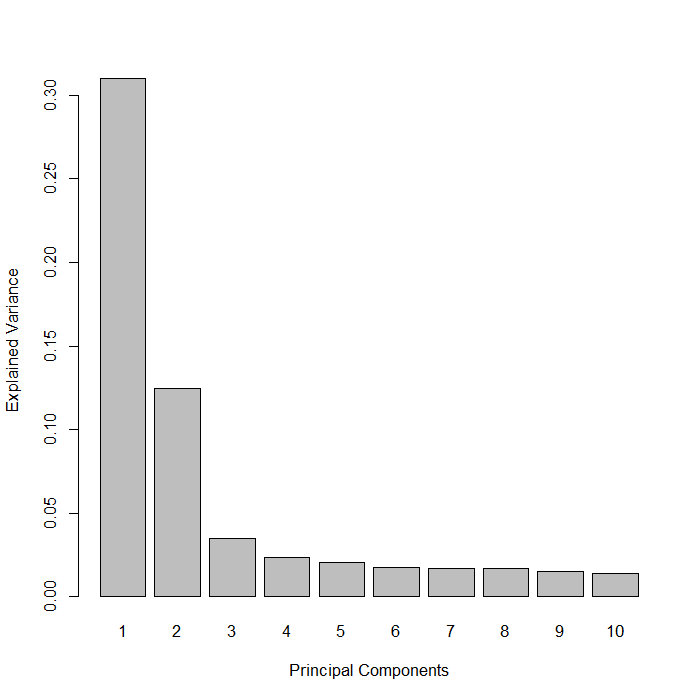FIGURE 1: Bar plot of the proportion of explained variance by each principal component yielded from a PCA. Below (in Figure 2), the samples can be seen projected onto the first two Principal Components . (a) shows the case where the repeated measures was not accounted for while (b) does control for this. Without a multilevel approach, the total explained variation decreases from 43% to 34%. In Figure 2a, the separation of each bodysite is distinct, but not the strongest. This is vastly improved when the multilevel framework is employed. The first component separates all three bodysites to a moderate degree, primarily discriminating between the stool and subgingival plaque.The second principal component separates the antecubital fossa bodysite from the others. # undergo PCA with 2 components diverse.pca.nonRM = pca(X, ncomp = 2, logratio = 'CLR') # undergo PCA with 2 components and account for repeated measures diverse.pca.RM = pca(X, ncomp = 2, logratio = 'CLR', multilevel = sample) plotIndiv(diverse.pca.nonRM, # plot samples projected onto PCs ind.names = FALSE, # not showing sample names group = Y, # color according to Y, title = '(a) Diverse.16S PCA Comps 1&2 (nonRM)') plotIndiv(diverse.pca.RM, # plot samples projected onto PCs ind.names = FALSE, # not showing sample names group = Y, # color according to Y legend = TRUE, title = '(b) Diverse.16S PCA Comps 1&2 (RM)')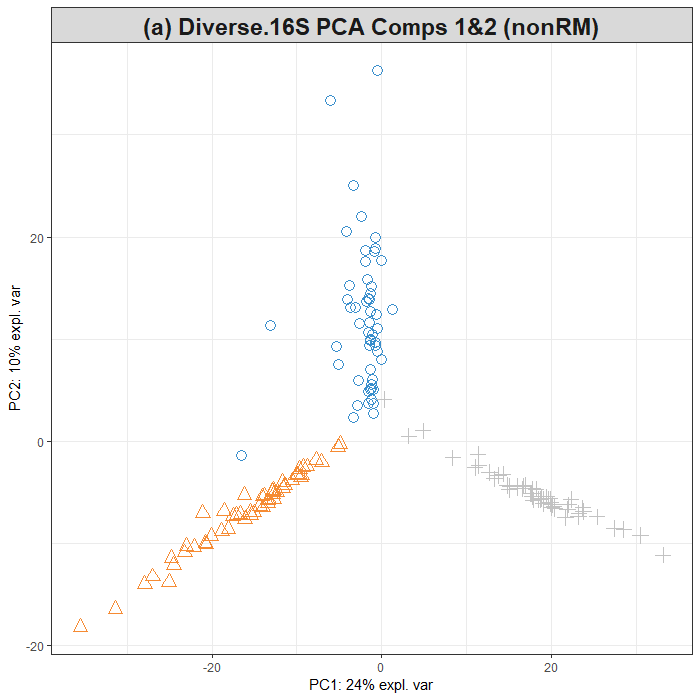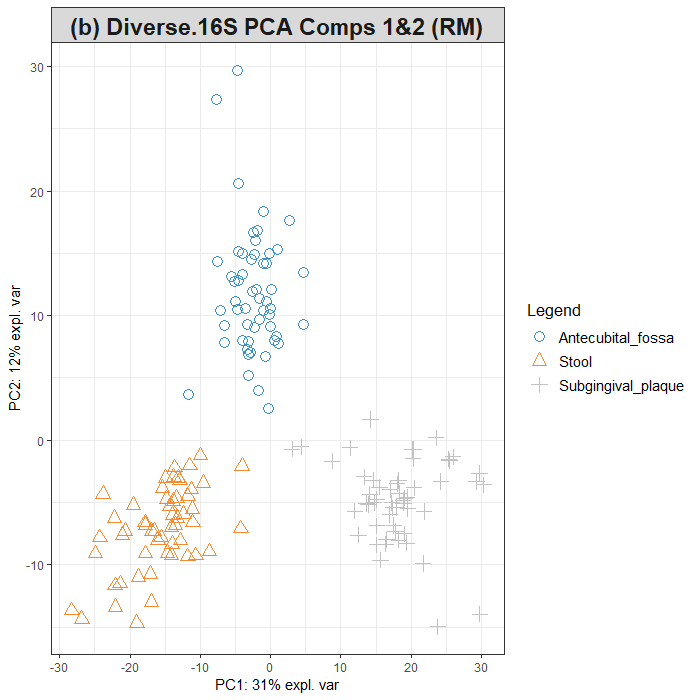FIGURE 2: Sample plots from PCA performed on the Diverse 16S OTU data. Samples are projected into the space spanned by the first two components. (a) depicts this when the repeated measures is not accounted for. (b) does use a multilevel framework. (‘RM’ = repeated measures) ### Initial sPLS-DA model The mixMC framework uses the sPLS-DA multivariate analysis from mixOmics . Hence, the next step involves generating a basic PLS-DA model such that it can be tuned and then evaluated. In many cases, the maximum number of components needed is k-1 where k is the number of categories within the outcome vector (y) – which in this case is 3. Once again, the logratio parameter is used here to ensure that the OTU data are transformed into an Euclidean space. basic.diverse.plsda = plsda(X, Y, logratio = 'CLR', ncomp = nlevels(Y), multilevel = sample)  ## Tuning sPLS-DA ### Selecting the number of components #### The ncomp Parameter To set a baseline from which to compare a tuned model, the performance of the basic model is assessed via the perf() function. Here, a 5-fold, 10-repeat design is utilised. To obtain a more reliable estimation of the error rate, the number of repeats should be increased (between 50 to 100). Figure 3 shows the error rate as more components are added to the model (for all three distance metrics). As this is a balanced dataset, the overall error rate and balanced error rate are the same (hence there seemingly being only one line on each set of axes in Figure 3). The plot indicates a decrease in the classification error rate (i.e. an increase in classification performance) from one component to 2 components in the model. The performance does not increase after 2 components, which suggests ncomp = 2 for a final PLS-DA model. Note that for the sparse PLS-DA we may obtain a different optimal ncomp. # assess the performance of the sPLS-DA model using repeated CV basic.diverse.perf.plsda = perf(basic.diverse.plsda, validation = 'Mfold', folds = 5, nrepeat = 10, progressBar = FALSE) # extract the optimal component number optimal.ncomp <- basic.diverse.perf.plsda$choice.ncomp["BER", "max.dist"]

plot(basic.diverse.perf.plsda, overlay = 'measure', sd=TRUE) # plot this tuning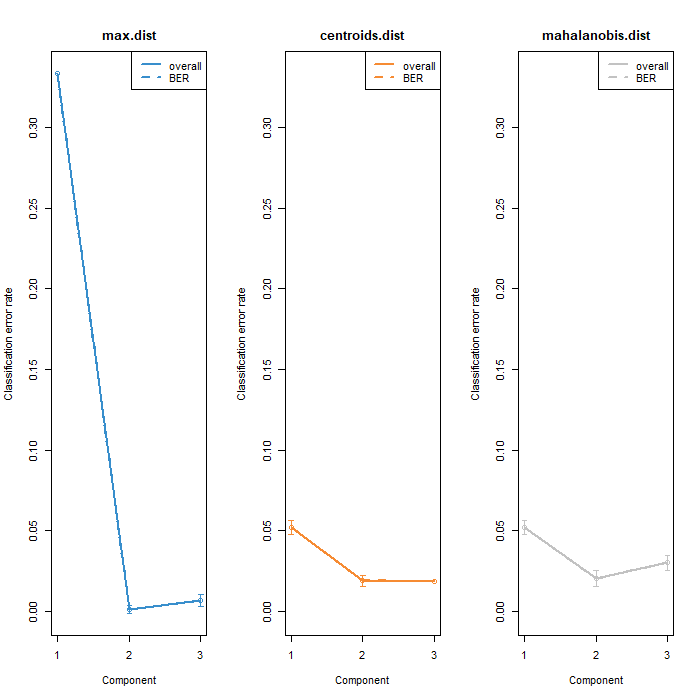FIGURE 3: Classification error rates for the basic sPLS-DA model on the Diverse OTU data. Includes the standard and balanced error rates across all three distance metrics.

### Selecting the number of variables

#### The keepX Parameter

Using the tune.splsda() function, the optimal number of components can be confirmed as well as the number of features to use for each component can be determined. Once again, for real analysis a larger number of repeats should be used compared to the 5-fold, 10-repeat structure used here. It can be seen in Figure 4 that adding a third component does not improve the performance of the model, hence ncomp = 2 remains valid. The diamonds indicate the optimal keepX values for each of these components based on the balanced error rate.

set.seed(5249)

grid.keepX = c(seq(5,150, 5))

diverse.tune.splsda = tune.splsda(X, Y,
ncomp = 3, # use optimal component number
logratio = 'CLR', # transform data to euclidean space
multilevel = sample,
test.keepX = grid.keepX,
validation = c('Mfold'),
folds = 5, nrepeat = 10, # use repeated CV
dist = 'max.dist', # maximum distance as metric
progressBar = FALSE)

# extract the optimal component number and feature count per component
optimal.ncomp = diverse.tune.splsda$choice.ncomp$ncomp
optimal.keepX = diverse.tune.splsda$choice.keepX[1:optimal.ncomp] plot(diverse.tune.splsda) # plot this tuning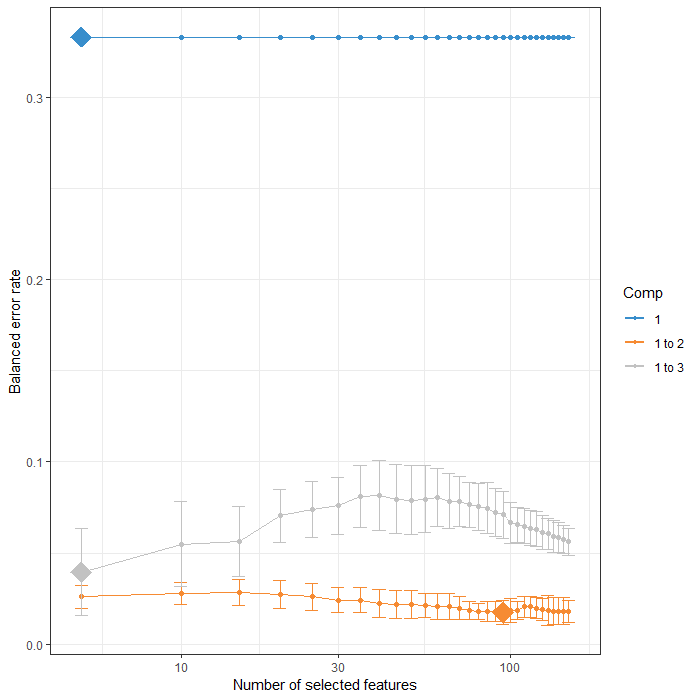FIGURE 4: Tuning keepX for the sPLS-DA performed on the Diverse OTU data. Each coloured line represents the balanced error rate (y-axis) per component across all tested keepX values (x-axis) with the standard deviation based on the repeated cross-validation folds. ## Final Model Following this tuning, the final sPLS-DA model can be constructed using these optimised values. diverse.splsda = splsda(X, Y, logratio= "CLR", # form final sPLS-DA model multilevel = sample, ncomp = optimal.ncomp, keepX = optimal.keepX)  ## Plots ### Sample Plots The sample plot found in Figure 5 depicts the projection of the samples onto the first two components of the sPLS-DA model. The subgingival plaque is adequately separated from the other two sites along the first component. The antecibital fossa and stool sites are better separated by the second component, though the overlapping confidence ellipses shows that this component is not the best at discriminating between them. Do no hesitate to add other components and look at the sample plot to visualise the potential benefit of adding a third component as the current separation of bodysites could do with improvement. plotIndiv(diverse.splsda, comp = c(1,2), ind.names = FALSE, ellipse = TRUE, # include confidence ellipses legend = TRUE, legend.title = "Bodysite", title = 'Diverse OTUs, sPLS-DA Comps 1&2')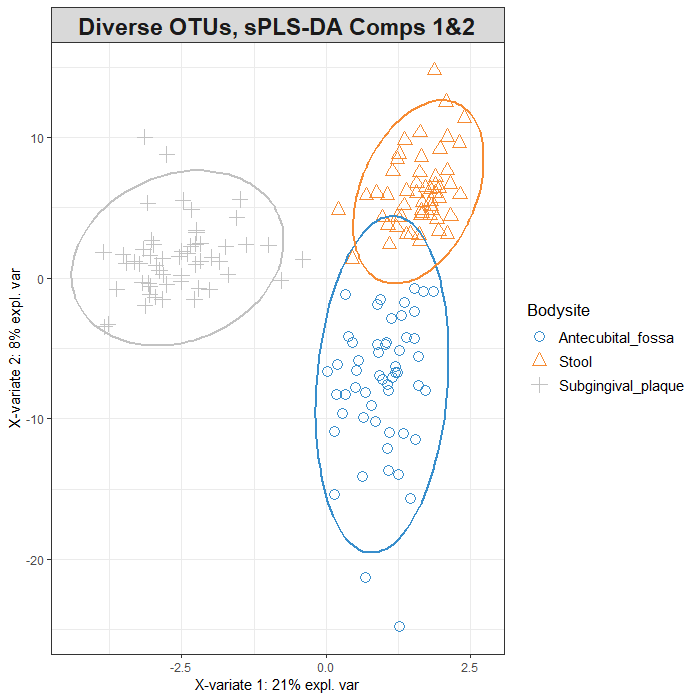FIGURE 5: Sample plots from sPLS-DA performed on the Diverse OTU data. Samples are projected into the space spanned by the first two components. Another way to visualise the similarity of samples is through the use of a clustered image map (CIM). Figure 6 shows some relationships between OTUs and certain bodysites. For example, the right-most cluster of OTUs seems to be positively associated with the subgingival plaque site – while the vast majority of other OTUs have a negative association with this same site. cim(diverse.splsda, comp = c(1,2), row.sideColors = color.mixo(Y), # colour rows based on bodysite legend = list(legend = c(levels(Y))), title = 'Clustered Image Map of Diverse Bodysite data')FIGURE 6: Clsutered Image Map of the Diverse OTU data after sPLS-DA modelling. Only the keepX selected feature for components 1 and 2 are shown, with the colour of each cell depicting the raw OTU value after a CLR transformation. ### Variable Plots Next, the relationship between the OTUs and the sPLS-DA components is examined. Note that cutoff = 0.5 such that any feature with a correlation vector length less than 0.5 is not shown. The three clusters of variables within this plot correspond quite well to the three bodysite clusters in Figure 5. Interpretting Figure 7 in conjunction with Figure 5 provides key insights into what OTUs are responsible for identifying each bodysite. For example, the cluster of 4 OTUs at the negative end of the first component (left side) in Figure 7 are likely to be key OTUs in defining the microbiome in the subgingival area. plotVar(diverse.splsda, comp = c(1,2), cutoff = 0.5, rad.in = 0.5, var.names = FALSE, pch = 19, title = 'Diverse OTUs, Correlation Circle Plot Comps 1&2')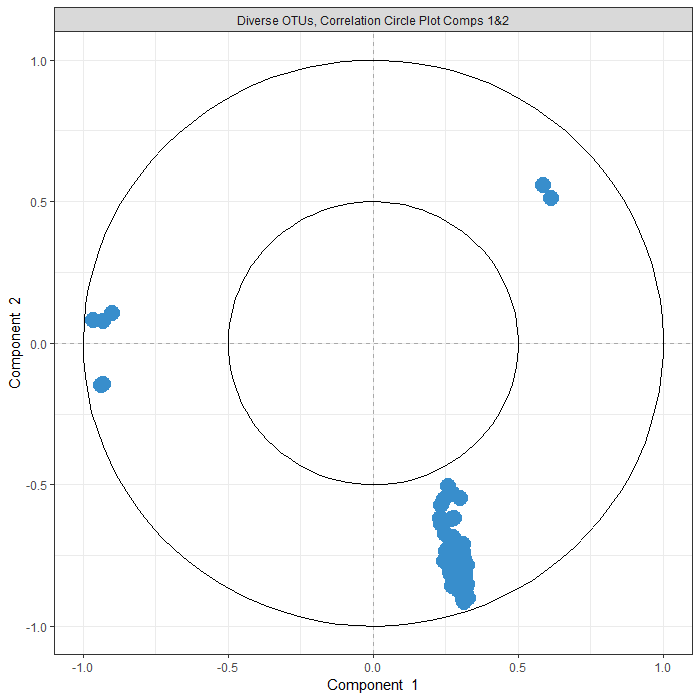FIGURE 7: Correlation circle plot representing the OTUs selected by sPLS-DA performed on the Diverse OTU data. Only the OTUs selected by sPLS-DA are shown in components 1 and 2. Cutoff of 0.5 used ## Evaluation of sPLS-DA The mixOmics package also contains the ability to assess the classification performance of the sPLS-DA model that was constructed via the perf() function once again. The mean error rates per component and the type of distance are output. It can be beneficial to increase the number of repeats for more accurate estimations. It is clear from the below output that adding the second component drastically decreases the error rate. set.seed(5249) # for reproducible results for this code, remove for your own code # evaluate classification using repeated CV and maximum distance as metric diverse.perf.splsda = perf(diverse.splsda, validation = 'Mfold', folds = 5, nrepeat = 10, progressBar = FALSE, dist = 'max.dist') diverse.perf.splsda$error.rate

## $overall ## max.dist ## comp1 0.33333333 ## comp2 0.01728395 ## ##$BER
##         max.dist
## comp1 0.33333333
## comp2 0.01728395


## OTU Selection

The sPLS-DA selects the most discriminative OTUs that best characterize each body site. The below loading plots (Figures 9a&b) display the abundance of each OTU and in which body site they are the most abundant for each sPLS-DA component. Viewing these bar plots in combination with Figures 5 and 7 aid in understanding the similarity between bodysites. For both components, the 20 highest contributing features are depicted.

OTUs selected on the first component are all highly abundant in subgingival plaque samples based on the mean of each OTU per body site. This makes sense based on the interpretations of Figures 5 and 7. All OTUs seleced on the second component are strongly associated with the antecubital plaque site.

plotLoadings(diverse.splsda, comp = 1,
method = 'mean', contrib = 'max',
size.name = 0.8, legend = FALSE,
ndisplay = 20,

method = 'mean', contrib = 'max',
size.name = 0.7,
ndisplay = 20,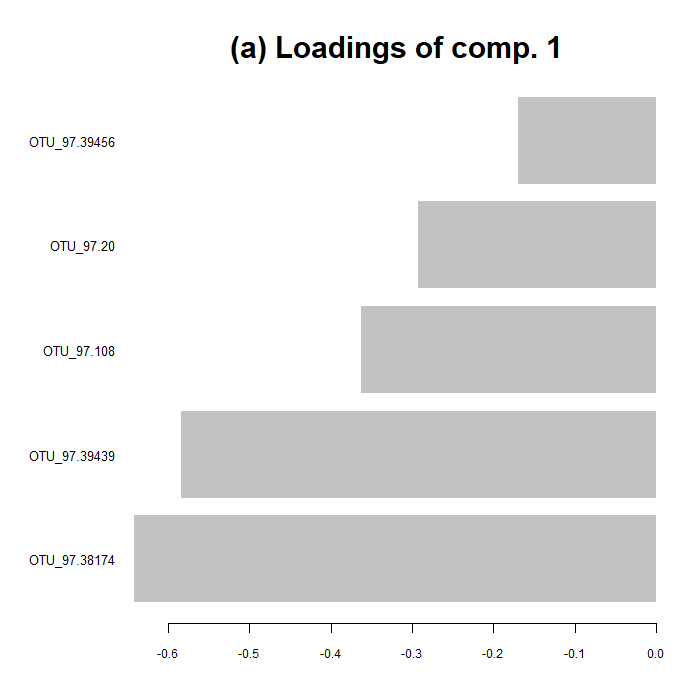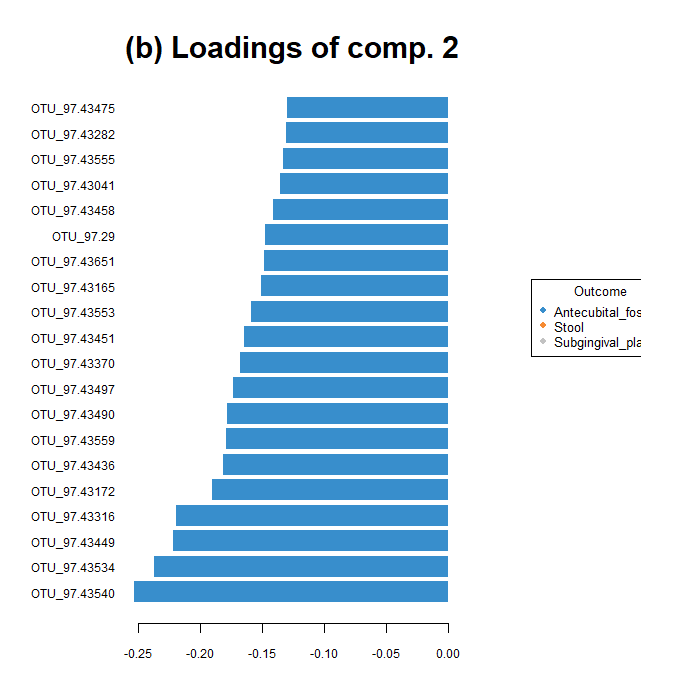FIGURE 9: The loading values of the top 20 (or 5 in the case of comp. 1) contributing OTUs to the first (a) and second (b) components of a sPLS-DA undergone on the Diverse OTU dataset. Each bar is coloured based on which bodysite had the maximum, mean value of that OTU.

To take this a step further, the stability of each OTU on these components can be assessed via the output of the perf() function. The below values (between 0 and 1) indicate the proportion of models (during the repeated cross validation) that used that given OTU as a contributor to the first sPLS-DA component. Those with high stabilities are likely to be the most important to defining a certain component.

# determine which OTUs were selected
selected.OTU.comp1 = selectVar(diverse.splsda, comp = 1)$name # display the stability values of these OTUs diverse.perf.splsda$features\$stable[][selected.OTU.comp1]

##
## OTU_97.38174 OTU_97.39439   OTU_97.108    OTU_97.20 OTU_97.39456
##         1.00         0.86         0.92         0.86         0.52